# Triple Bonus Roulette System

Congratulations! You have acquired a new winning Roulette system, one that will give you lots of fun and excitement playing Roulette, while making profits.

When one places outside bets, we all know it is a nuisance to have one zero coming up and swiping away all your bets. This is really where the house advantage of 5.26% comes from. Triple Bonus Roulette will turn the zeros against the casino and will convert the house advantage into yours.

The system is based on Dozens or Columns, where the payoff is 2 to 1.

The system will require you to bet on all three Dozens or Columns at the same time by varying the amounts of your bets from one sequence to another. We will first identify the winning concepts of the procedures and build progressively in a step by step approach towards the final system.

Most of us are acquainted with the D'Alenbert system, which is based on starting with one unit and increasing your bet by one unit when you win and decreasing it by one when you lose. When we bet on the Dozens, we will apply this concept with the following modifications: When we lose our bet, we will increase it by one unit. When we win our bet, we will decrease it by two units instead of one. Also, we will not start betting with one unit, but with 20 units.

Now, don't be alarmed, as at this stage, we are only building our system by talking about its winning concepts. In the final system, you will never have to place more than 25 units on the table at a time.

Now, let's see why this kind of progression generates a unit profit per spin in average. The probability of a Dozen to come up is 1 out of 3 if we ignore the zeros for a moment. If we don't ignore the zeros, this probability is exactly 12 out of 37 or 38 depending if we are on a single zero European Roulette or a double zero American Roulette respectively. The probability of a zero to come up is 1 out of 37 on a single zero European Roulette and 2 out of 38 or 1 out of 19 on a double zero American Roulette. Based on those probabilities, we can assume that in average one Dozen will win once in three times and a zero will show up once every 37 spins on a European Roulette and once every 19 spins on an American Roulette making the Dozen lose. Based on the above progression, if you place 20 units on a Dozen and you lose twice before it shows.

Up, you will be ahead by 3 units at the end of 3 spins:

• Bet 20, you lose, you are at -20.
• Increase by 1, bet 21,you lose, you are at -41.
• Increase by 1, bet 22, you win, you cash 66, you have put so far 63 in action, your net profit is +3 units.

The above example was for a sequence of losing twice first, then winning once.

The order of winning and losing can be arbitrary.

The following few tables will illustrate this concept:As you can see, it doesn't matter when the losing or the winning bet occurs, the outcome is 3 unit profit at the end of the progression: increase by one when you lose and decrease by 2 when you win, as long as you don't start with only one unit. If you had started your progression with one unit as the classic d'Alambert requires, only the first table above would have generated 3 unit profit, and in the other 2 tables you wouldn't be able to decrease your bet by 2 units, as you would end up with 0 or -1. You would then bet 1 unit and your overall net profit would decrease by 1 and 2 units in the second and third table respectively.

The following three tables illustrate the classic d'Alenbert system: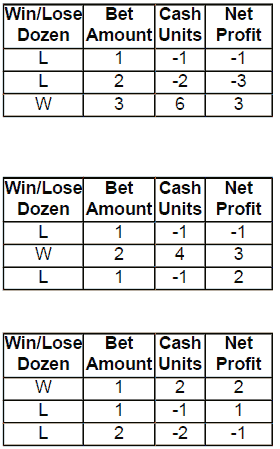As you can see, starting with one unit at the beginning of the progression, does not generate a 3 unit profit every time. This is why we begin our progression with a unit amount greater than 3. The unit value 20 was chosen to be able to pursue with the progression in case of an occurrence of a winning streak, where you would be able to decrease your bet by 2 units every time you win the bet.

This means that you can apply this progression over many spins and you will end up with 3 unit profit in 3 spins or 1 unit profit per spin in average, as long as you win once out of 3 times on average.

The following table will illustrate this fact: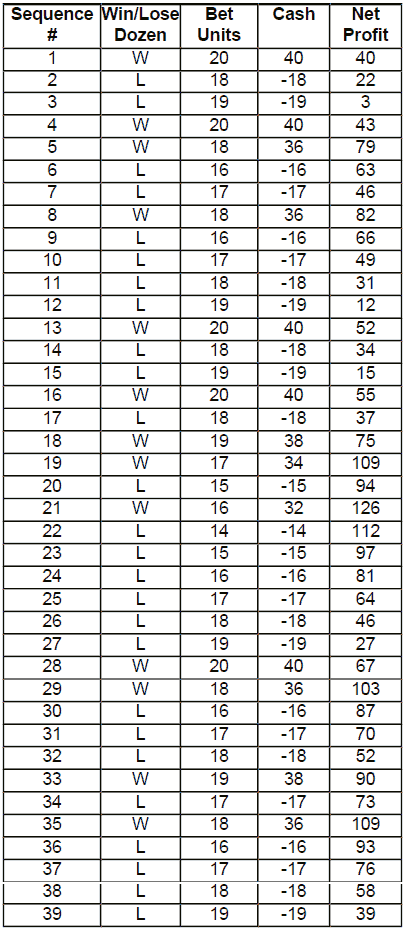We won13 out of 39 bets (1 out of 3 on average) and we ended up with a 39 unit profit averaging one unit profit per bet. This works over a long run even if you have multiple winning and losing streaks, as long as we win one third of all our bets and this is compliant to the probability of the Dozens or Columns.

This can already be used as a system in itself. It will perform well on a single zero wheel. We could assume that the bet in sequence 38 in the above table was a zero, causing the Dozen to lose. The zero would be coming up once every 37 spin statistically and we would still be winning one third (almost) of our bets.

The question would then become, which Dozen do you decide to bet on? My experience has been that usually one Dozen performs well, another Dozen not so well, and a third performs OK in average. If you stick to one Dozen (Dozen 1, 2 or 3), you may end up choosing the Dozen that does not perform that well and end up in losses. If you place your bets on any Dozen chosen at random, the results can be very unpredictable as you are relying on your luck, although in the long run, you should be OK if you win one third of all your bets.

To make our decision easier and also to triple our profits we will be betting on all 3 Dozens or Columns at the same time. This way we will be averaging one unit profit per Dozen or 3 units profit on all 3 Dozens per spin. Please remember we are still building our winning system, and we are presently still talking about its concept. I will let you know when it will become final. So, do not use the following table as illustrated.

The following table (which is not the system yet) will show what would happen if we would bet on all 3 Dozens at the same time, assuming at first that there are no zeros. One of the three Dozens is surely to win on every spin:

Table 1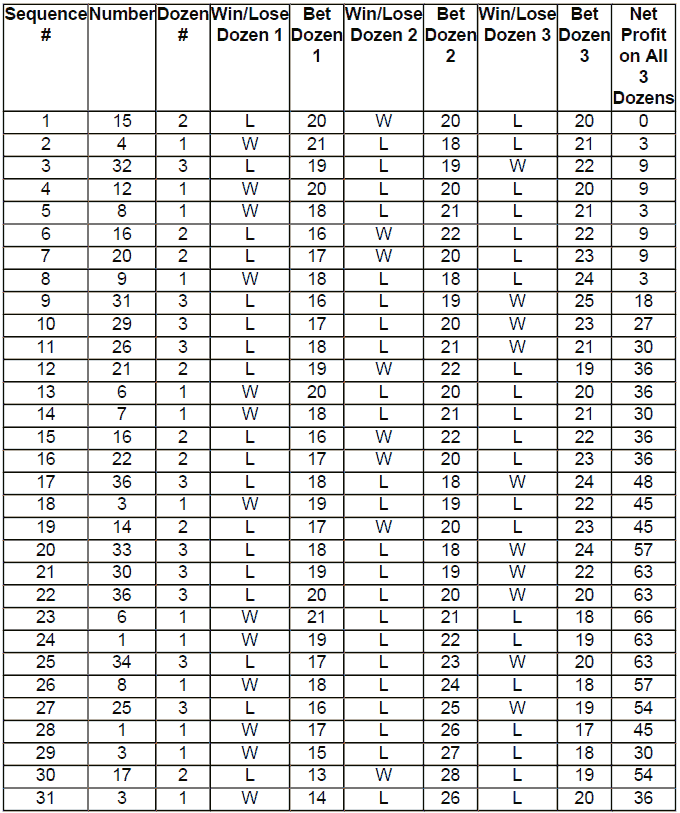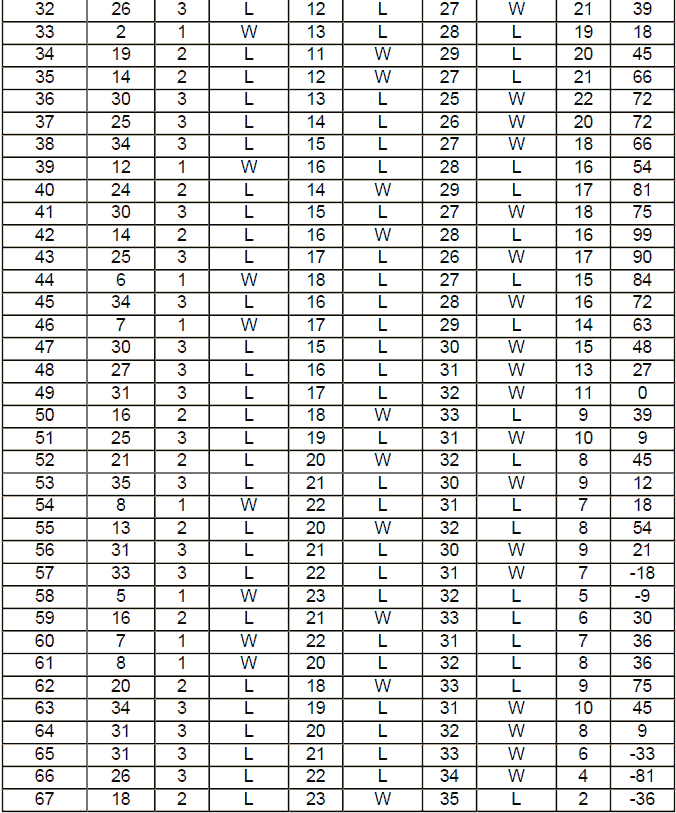Now let's explain what goes on the above table.

Here are the first 10 spins for convenience: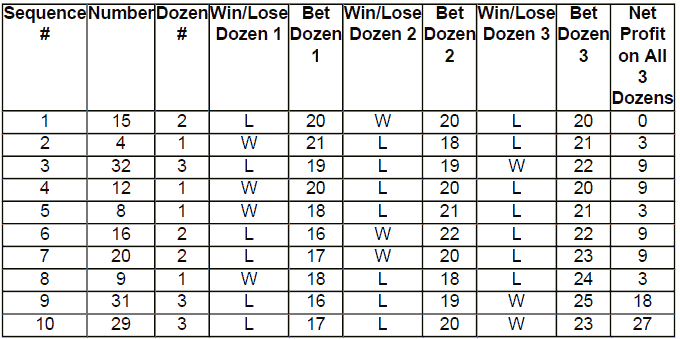The very first bet in Sequence 1 is really a virtual bet to see which Dozen will win. Otherwise you would never place 20 units on each Dozen. It's like not betting. Or even worse, a zero could come and wipe out your 60 units off the table. Number 15 or Dozen 2 is the outcome of Sequence 1. As we have started to bet with 20 units, and as Dozen 2 won, we bet two units less on Dozen 2, that is 18 units and one unit more on each Dozen 1 and Dozen 3 going to 21 units.

Number 4 or Dozen 1 comes up in sequence 2, we win the 21 unit bet and lose the other 21 and 18 unit bets. Winning the 21 unit gives us a profit of 42 minus the 21 and 18 units = 39 of the other two losing Dozens gives us an overall profit of 3 units.

Now, as we won on Dozen 1 and lost on Dozens 2 and 3, we decrease our bet by two units on Dozen 1 going from 21 to 19 and increase our bets on Dozens 2 and 3 by one unit going to 19 and 22 respectively in sequence 3. This time, Dozen 3 wins and we cash 44 units from Dozen 3 and lose the two 19 units from Dozen 1 and 2, profiting for this sequence 44-38=6 more units, bringing our overall net profit to 9 units.

Following the remaining spins shown in the above table, the procedure goes on and on, increasing our bet by one unit on the two Dozens we lose, and decreasing by two units on the Dozen we win, giving us steady profits if no drastic losing streak on any Dozen occurs.

This looks rosy, but it's not. First, we have assumed that there are no zeros.

Secondly, we are betting close to 60 units on every sequence. If a zero would show up every say 20 sequences, we would be losing 60 X 5 = 300 units within 100 spins. There goes our 279 unit profit (see Table at sequence 100) down the tube. Well, don't despair. I have a very ingenious solution and it is the beginning of the foundation of our system.

Let's take a look at sequence 5 in Table 1 above. We are betting 18 units on Dozen1, 21 units on Dozen 2 and 21 units on Dozen 3. This is placing a total bet of 18 + 21 + 21 = 60 units. If Dozen 1 wins, we cash 36 units on Dozen 1 and lose 2 X 21 = 42 units on the other two Dozens, losing 36-42=6 units in this sequence.

If either Dozen 2 or 3 wins, we cash 42 units on the winning Dozen and lose 18 + 21 = 39 units on the other two Dozens, profiting 42-39=3 units. But, the whole sequence is equivalent to betting 3 units on Dozen 1 and 6 units on each Dozen 2 and 3. If you calculate either case of winning or losing Dozen, you will see that the result is identical.Now, this will lead us to the actual formula we will us in our system. What we will do is to subtract the smallest bet unit amount of the three Dozens from the other two. The result will be the following: no bets will be placed on the Dozen with the smallest unit and the other two Dozens will have the bet amount equal to their difference from the smallest unit.

We will illustrate this with an example. Let's take a look at sequence 79 on Table 1 above. It shows that we need to place 20 units on Dozen 1, 29 units on Dozen 2 and 11 units on Dozen 3. The smallest unit amount is on Dozen 3 (11 units).

We subtract 11 from the amounts of Dozen 1 and 2. We get 20 - 11 = 9 units for Dozen 1 and 29 - 11 = 18 units on Dozen 2. In sequence 79, Dozen 1 is the winning one. According to our formula, we now should bet 9 units on Dozen 1, 18 units on Dozen 2 and nothing on Dozen 3, instead of 20 units on Dozen 1, 29 units on Dozen 2 and 11 units on Dozen 3. And we get exactly the same result when Dozen 1 wins, that is we cash 18 units profit on Dozen 1 and lose the 18 units on Dozen 2, breaking even. Therefore the overall profit remains 153 as in the previous sequence 78.

The advantage of this formula is great. Because now, you are placing a total of 9 + 18 = 27 units on the table, instead of 20 + 29 + 11 = 60 units. If a zero would show up, you would lose only 27 units instead of 60.

Let's take another example to make sure this is understood, as it is extremely important.

In sequence 62, we were supposed to place 18 units on Dozen 1, 33 units on Dozen 2 and 9 units on Dozen 3. The smallest amount is on Dozen 3. Subtract 9 units from 18 and 33, we get to place 18-9=9 units on Dozen 1, 33-9=24 units on Dozen 2 and 9-9=0 or no bets on Dozen 3. Dozen 2 wins this sequence cashing 48 units minus the 9 units on the losing Dozen 1, profiting 39 units for the sequence, bringing our overall net from 36 to 75 units.

It's not easy to make all those calculations and equivalent bets when you are under the pressure of betting. It's best to take a pad and a pencil and do the arithmetic on every sequence.

So you will scribble down your next 3 bets on 3 Dozens and write next to each Dozen the equivalent amount. So your pad will look something like this:

Dozen 1              Dozen 2              Dozen 3

16->(-16=0)       19->(-16=3)       25->(-16=9)

This was for sequence 9 in Table 1. Smallest bet is 16 units. Dozen 1 goes to no bet. Dozen 2 goes to 19-16= 3 units. Dozen 3 goes to 26-16=9 units.

With a little practice you will get the feel of it very quickly. So in reality you keep track of what you were supposed to bet, having started from 20 units on each Dozen and actually bet the equivalent reduced bets, which will give identical results, saving on the amounts when the zeros show up.

To help you understand the method, let’s now repeat the first 10 sequences from Table 1, except this time the table shows the units you would have actually bet:

That makes a tremendous difference, doesn't it? Now you work with a considerably smaller bankroll, betting greatly reduced amounts and have the exact same results. Additionally, your losses are not as heavy when a zero comes.

We are still making a profit of 3 units per spin on average. If we get several zeros, i.e. more than the frequency of one every 19 spins, then we would be no longer making this profit. The house edge would kick in and we would start losing money.

The following solution will bring us to the last stage of building our system. If we are making a profit of 3 units per spin with the above progression (increase by one when you lose, decrease by 2 when you win), we can afford to place one unit on each zero. If there are two zeros, we would be paying a commission of 2 units per bet. This will reduce our profit to 3-2=1 unit per spin on average. If there is only one zero, we would be paying a commission of 1 unit per spin, reducing our profit to 3-1=2 units per spin. However, on American wheels, the frequency of zeros appearing will be once in every 19 spins on average. On European wheels, the frequency of the zero showing up will be once in every 37 spins in average.

For every bet we use the progression as shown in Table 1, but the difference is that we place one additional unit on each zero. Now we are making only 1 unit profit per spin on average. But, every time a zero comes, we get a 35-unit increase in our net profit! This reverts the house advantage to yours. We will illustrate how this works in Table 2 below.

Please note that Table 2 is already taking advantage of the reduced bets by selecting the smallest bet of all 3 Dozens, subtracting the amount from the other two Dozens and calculating the Sum of all bets placed on the table.

D1 W/L, D2 W/L, D3 W/L columns indicate if Dozen 1, 2 or 3 are winning or losing respectively. Dozen 1 is for numbers 1-12. Dozen 2 is for numbers 13-24.

Dozen 3 is for numbers 25-36. Dozen 0 is designated for 0 or 00.

Table 2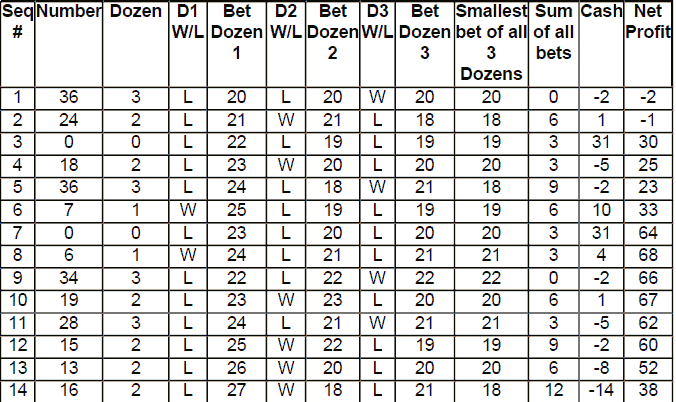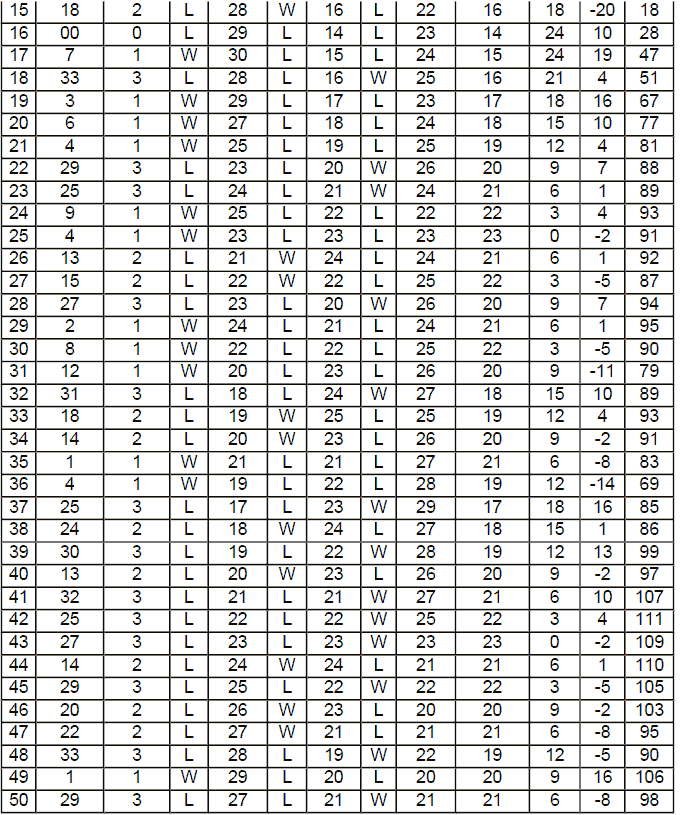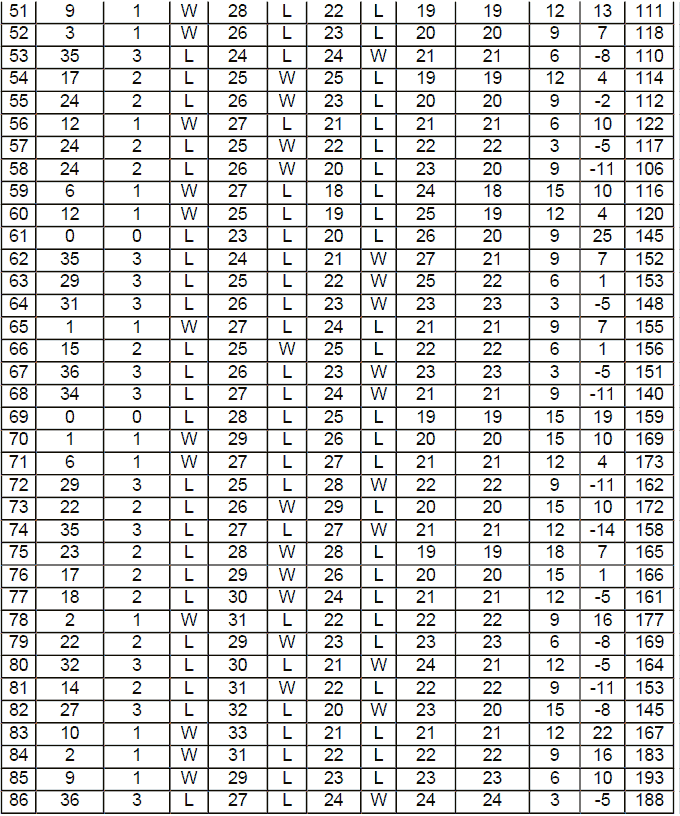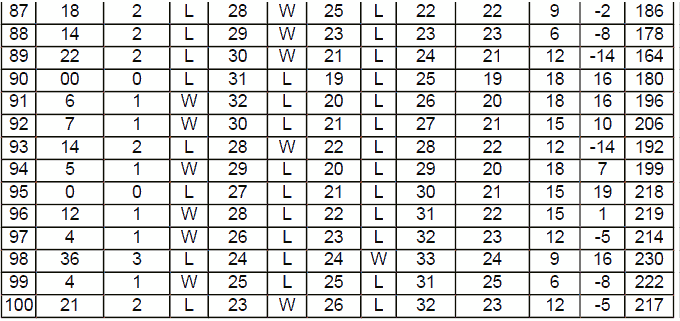So, Table 2 above illustrates the ultimate system and it is in its final shape.

There are also some money management strategies that I would like to talk about in a moment.

But, first, let us take another look at Table 2 above, and see what is going on.

Here are the first 10 sequences again for convenience: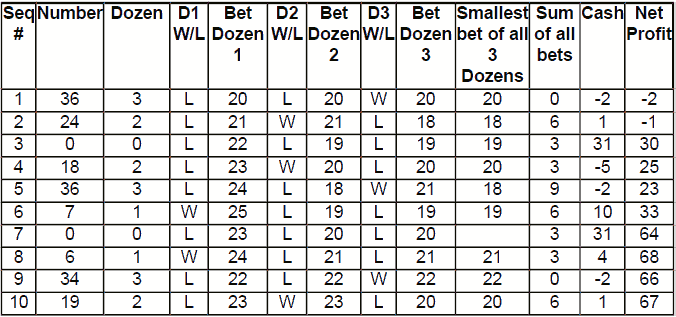In sequence 1, since all bets on each Dozen is 20 units, there are no bets placed on Dozens, except for 2 units on each zero. The zero does not come up, we are even on the Dozens. Our net is -2, the two units we lost on the zeros.

Since number 36 (Dozen 3) comes up in sequence 1, we decrease our 20 unit bet to 18 units for Dozen 3 and increase our 20 unit to 21 units on each Dozen 1 and Dozen 2. Now, this is equivalent of betting 3 units on each Dozen 1 and 2 and placing no bets on Dozen 3. We have simply subtracted 18 (the smallest bet) from each 21. Therefore the sum of all bets is 3 + 3 = 6 as shown on Table 2.

Number 24 comes up, Dozen 2 wins and we cash 3 units on Dozen 2. But remember we have placed 2 units on the zeros. So, we cash 3-2=1 unit only.

Our net becomes -1 unit.

In sequence 3, we decrease our bet by 2 units on Dozen 2 from 21 to 19 units, since it won on sequence 2. We increase our bets on Dozen 1 and 3 by 1 unit: on Dozen 1 from 21 to 22 and on Dozen 3 from 18 to 19. This is equivalent of betting 3 units on Dozen 1 (22-19) and not betting on Dozens 2 and 3 (18-18).

Therefore, the sum of all bets is 3 for this sequence. And, surprise, a zero comes up. We cash 36-2=34 units on the zero and lose the 3 units we have bet on Dozen 1, cashing overall 34-3=31 units as Table 2 shows. Our overall profit jumps from -1 to +30 units!

In sequence 4, we need to increase all three Dozens by 1 unit, since they all lost. Increasing all three Dozens by 1 unit doesn't change the amount of bets from the previous sequence 3 according to our equivalence formula, as the difference of the smallest and the other two bets will be the same, that is 3 units on Dozen 1 and nothing on the other 2 Dozens. (Right?) So, the sum of all bets is 3 again. Number 18 (Dozen 2) comes up. We lose our 3 unit bet on Dozen 1 and 2 units on the zeros. So we are down by -5 units, bringing our net to +25 units.

So, you must have already the idea on how the system works and how well it performs. Every time you hit a zero, you rejoice as you get a bonus of 34 units on top of your working triple Dozen system. During the simulation of Table 2, we hit the zero 7 times on sequences 3, 7, 16, 61, 69, 90 and 95 within 100 spins, which is normal considering the odds of twice within 38 spins. During those sequences, the sum of all bets were 3, 3, 24, 9, 15, 18 and 15 units respectively.

If a zero would hit without using the zero coverage strategy, we would have lost 3 + 3 + 24 + 9 + 15 + 18 + 15 = 87 units for those sequences. Instead we made a profit of (34 X 7 - 87 =) 151 additional units. Without the zero coverage strategy our overall profit would have been 217 - 151 = 66 units at the end of 100 sequences.

I will show you now how your bets would be placed on the table referring to the results of Table 2. Then I will provide you with an empty template that you can take with you when you go to the LIVE casino or when you play on-line.

Here are the first 10 bets again: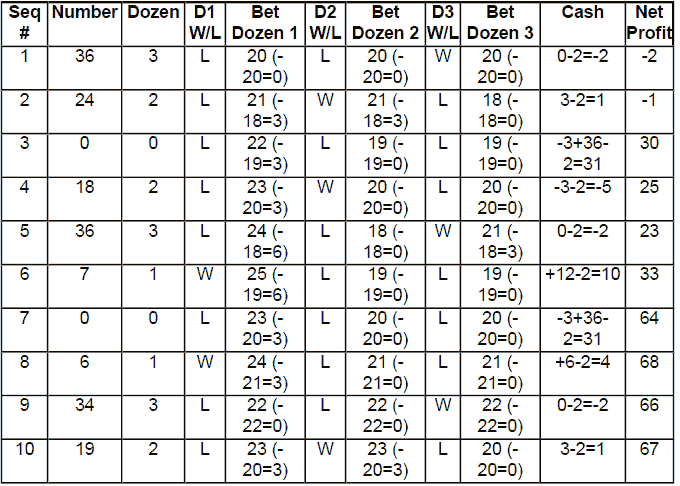In the above table, we perform the little arithmetic, which shows us how much to bet on each Dozen. The arithmetic consists of reducing the smallest Dozen bet from each amount. We have a "-2" in cash column showing our bets on the zeros.

As you can see we are betting nothing on the Dozens in sequence 1 (0,0,0), but betting one unit on zero and one unit on the double zero. Therefore our net profit is -2.

In sequence 2, we reduce the winning Dozen (3) of sequence 1, by two units and increase the other 2 Dozens by one unit. But we don't bet 21, 21 and 18 on the three Dozens respectively, but only 3 units on Dozen 1, 3 units on Dozen 2 and nothing on Dozen 3. Dozen 2 wins, we cash 6 units profit on Dozen 2 minus the 3 units we bet on Dozen 1 and minus the two units we placed on the zeros, giving us 1 unit profit overall for this bet.

In sequence 3, we place 3 units on Dozen 1 and nothing on the other two Dozens and always one unit on the zero and one unit on the double zero. The zero comes, we lose our 3 unit bet on Dozen 1 but gain 36 on the zero. Overall, we make 36 minus 3 and minus the 2 units we bet on the zeros giving us 31 units profit for that bet.

In sequence 4, we place 3 units on Dozen 1 and nothing on the other two Dozens, as the arithmetic shows. This time Dozen 2 wins and we lose this 3 unit bet including the two units on the zeros.

In sequence 5, we place 6 units on Dozen 1 and 3 units on Dozen 3 (and of course, two units on the zeros). Dozen 3 wins, we break even on the Dozens and lose the two units on the zeros. Therefore, our net goes down by 2 units.

In sequence 6, we place 6 units on Dozen 1 (25-19) and nothing on the other two Dozens. And Dozen 1 wins. We make 12 unit profit on Dozen 1 minus the two units on the zeros. Our bankroll goes up by 10 units (from 23 to 33).

In sequence 7, we place 3 units on Dozen 1 and nothing on the other two Dozens and two units on the zeros. A zero comes, we make 36 units on the zero, minus our 3 unit bet on Dozen 1 minus the 3 units on Dozen 1 giving us 36-2-3=31 unit profit for that bet.

In sequence 8, we virtually increase all the bets by one unit, since zero came, all Dozens have lost. But those are all imaginary bets. In reality we bet only the difference of what the progression shows minus the smallest bet on that sequence. Since the smallest bet is 21 in that sequence, we reduce it from all the other Dozens. Dozen 1 goes from 24 to 3 (24-21), Dozen 2 goes to nothing (21-21) and so does Dozen 3 (21-21=0). So the only bets we place on the table is the 3 unit on Dozen 1 and the two units on the zeros, greatly reducing the amount of units we place on the table: 5 units instead of (24+21+21+2=68) units.

And the results are completely identical.

The following is an empty template you can take with you in order to write down the number that comes up on the wheel, the Dozen number, the progression that the system requires, the converted bets that you actually place on the table. You can write a "W" next to the Dozen, which wins. This will determine your next bet on the Dozens. The one that wins, will have two units less on the next bet. The two other Dozens that lose will have one unit more on the next bet. It's good to practice and get used to the system before you use it for real.

Casinos allow usage of pads and pens freely. They think you are keeping track of your scores.

The next page contains the template you will have with you. Print out a few copies of it to use it for real and for practice.

Here, let us practice together.

You come to the Roulette table, the first thing you do is place one unit on the zero and one unit on the double zero. You don't need to be concerned what was on the scoreboard, nor what the previous number was. Your first bet has nothing on the Dozens. But you still write 20 à 0 under the column Bet Dozen 1 and 20 à 0 under the column Bet Dozen 2 and 20 à 0 under the column Bet Dozen 3 for the sake of the system, as if you were betting 20 units on Dozen 1, 20 units on Dozen 2 and 20 units on Dozen 3. And subtract this 20 from all 3 Dozens giving 0.

Say number 12 comes up. You write a "W" under D1 W/L column in sequence 1. You only lose the two units placed on the zeros. So the first line of your template will have the following written: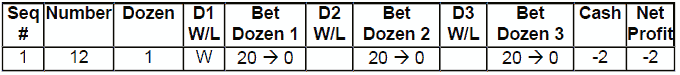What will your next bet be? Dozen 1 won, so it goes to 18 (decrease by 2 units).

Dozen 2 and 3 lost. They both go to 21 (increase by 1 unit). The smallest of 18, 21, 21 is 18. Subtract 18 from the three virtual bets on the Dozens (18, 21, 21) you will get 0, 3, 3 respectively for each Dozen. So you will write 18 à 0 under Bet Dozen 1, 21-18à3 under Bet Dozen 2 and 21-18à3 under Bet Dozen 3. And you place no bets on Dozen 1, 3 units on Dozen 2 and 3 units on Dozen 3, 1 unit on the zero and 1 unit on the double zero.

So your second line will look like the following so far: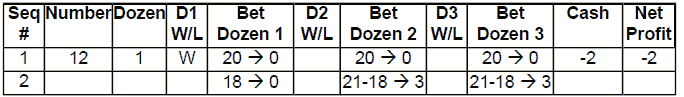Now 35 comes up, Dozen 3 wins. You write the Dozen number (3) under the column Dozen. You write a "W" under column D3 W/L to indicate that Dozen 3 won. You cash 6 units on Dozen 3, you lose your bet on Dozen 2 and you lose your 2 units on the zeros. So you cash 6-3-2=1 unit. You profit goes to -1 unit.

So, you record all this on your template: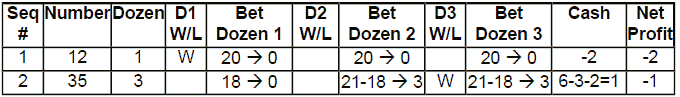As Dozen 3 wins, its virtual bet goes down 2 units from 21 to 19. As Dozens 1 and 2 lost, their virtual bets go up by 1 unit from 18 to 19 for Dozen 1 and from 21 to 22 for Dozen 2. The smallest bet unit of those three bets 19, 22, 19 is 19.

We subtract 19 from all 3. We register for Dozen 1: 19-19à0. For Dozen 2 we register 22-19à3. For Dozen 3 we register 19-19à0. So we bet only 3 units on Dozen 2 and nothing on the other Dozens. And of course one unit on the zero and one unit on the double zero. Your template looks now like the following: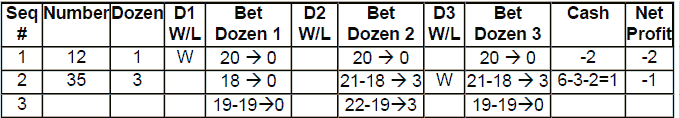Number 22 comes up. Dozen 2 wins. You mark W under D2 W/L. You cash 6 units on Dozen 2 and lose the two units on the zeros. Marking all this down, your third line looks: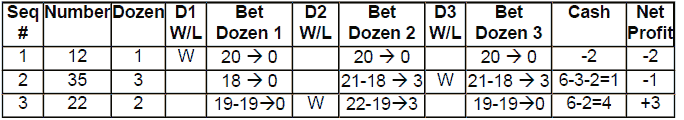Template

 Seq # Number Dozen D1 W/L Bet Dozen 1 D2 W/L Bet Dozen 2 D3 W/L Bet Dozen 3 Cash Net Profit

We can see already that by even distribution of 3 dozens winning at different times, we will make a profit of 3 units in 3 spins in average, thanks to the virtual units following the system's progression converted to much smaller units using the equivalence formula.

Now, some money management techniques. According to how the system is performing, we may want to use some variations of the above betting scheme.

One good money management system would be to stop the progression every time you hit a zero and start it over again from the very beginning with 20 units on each Dozen, regardless of where you were during this progression. This way, you capitalize on your sudden 34 unit increase, put your winnings in your pocket and start the progression over again as if it was a new day or a new table.

Another method would be to stop the progression if the sum of your bets reach 34 units. Otherwise, if you hit a zero, you would no longer be making any profit on that sequence.

Sometimes, you have long losing streaks on a particular Dozen. If this happens, a good method is to stop the increase of units on that Dozen and to either exclude that Dozen from your bets or reset it to its initial 20 unit bet. A long losing streak of a Dozen is the one losing more than 10 times in a row.

Ultimately, you can use my rule of thumb of money management: to quit the whole progression once you are ahead by 100 units or if you have lost 50 units.

This way, on good times if you win 6 times and lose 4 times, you will be ahead by (100 X 6) - (50 X 4) = 600 - 200 = 400 units and on bad times, if you win 4 times only and lose 6 times, you will still be ahead by (100 X 4) - (50 X 6) = 400 - 300 = 100 units.

Wishing you all the best and enjoyment of Roulette to the utmost.

Izak Matatya

## Play the Best LIVE Dealer Casinos### Lab Casino

100% up to €100### Casino Planet